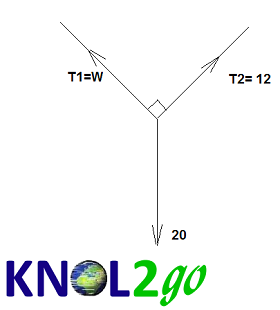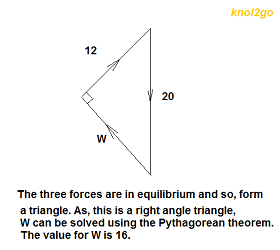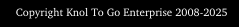Forces in Equilibrium

When forces are in equilibrium, the resultant force is zero. According to Newton's Second Law, a force on an object can be equated to the product of its mass, m, and acceleration, a. Hence, if the mass of the object, m, is not destroyed, the acceleration of the object, a, must therefore be equal to zero. The object is either stationary or moving at a constant velocity.

As
forces are vector quantities, they can be represented with the use of arrows. When three forces are in equilibrium, they follow the triangle of forces rule which states that the three arrows can form (make) a closed triangle. The direction of the arrows follow one another, as shown in the diagram below. The triangle of forces rule, for instance, can be used to solve many textbook questions, including the ones involving the weight(s) required in a pulley system which is in equilibrium.

Besides the triangle of forces rule, questions involving
forces in equilibrium can also be solved using parallelograms and/or trigonometry.Daya Seimbang

.